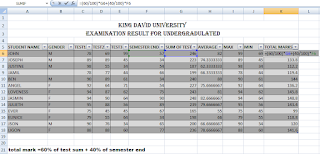# How to enter data in Excel sheet

How to fill Microsoft excel data in excel sheet

Hi today am here to discuss with you how you can fill your information in excel sheet

Follow step by step guide how to add the data in excel.

In order fill the data in excel sheet you must know first how to can you see Microsoft program and how can open this program.

First step am explain how you can open Microsoft excel in your computer.

To open Microsoft excel in your computer

1. open Microsoft excel by keyboard

2. open Microsoft excel by use mouse

To open Microsoft office excel by keyboard.

1. Start by clicking window button in your keyboard.

How to know window button?

The window button is a button which lies between ctrl button and alt button found left side down the keyboard. After click this button the window menu will display go to the search box and click all programs. After click it then open Microsoft office. Inside the Microsoft office choose Microsoft excel by click it to open. After click it the Microsoft excel will open. Method two

2. To open Microsoft excel by using mouse Point the window button by using mouse pointer left side down the monitor screen. After clicking button the window menu will display then point search box by mouse pointer and type the word ‘’excel’’ after type the word excel the Microsoft excel program will display as first program in list click to open. By clicking it the program will open, the active cell will be cell A1 and active sheet will be sheet one these will be shown by default.After open Microsoft

excel now you can start to fill your data in excel sheet as required see the sheet below

After filling information as shown above you can merge and center the title to make it clear.

to merge the cell in the excel you select all cell you need to be in one cell select from A2 to K2 after selecting these cell click home menu in excel then click merge and center  after click button  the button will shift to center of your work .

also you can bold the title of your work by selecting all the words that you need to bold then under the home menu click B button the B means bold  or you can use shortcut key in keyboard  CONTROL B is the shortcut key of bold.

Then format the worksheet as required. The worksheet will seen as picture below

Now am sharing how to calculate Sum of test, average, maximum, minimum and total marks

SUM OF TEST

To find sum of test, first method click the cell that required displaying the answer after click write the sum formula. The formula will be =SUM(C6:E6) Then click enter or ok where by C6 to E6 this means that all cell  from test 1 to test 3 will include in the sum when using this formula.

The second method you can use to find sum is by using auto sum. To use auto sum us as follow  click the cell required to display answer after click it select all the cell which you need to find sum then in choose the summation symbol in upper left side in the excel program then click sum

AVERAGE OF TEST

To find AVERAGE of test, first method click the cell that required displaying the answer after click write the AVERAGE formula. The formula will be = AVERAGE (C6:E6) Then click enter or ok where by C6 to E6 this means that all cell  from test 1 to test 3 will include in AVERAGE when using this formula. The second method you can use to find AVERAGE is by using auto sum. To use auto sum us as follow  click the cell required to display answer after click it select all the cell which you need to find AVERAGE then in choose the summation symbol in upper left side in the excel program then click AVERAGE

MAXIMUM OF TEST

To find Maximum of test, first method click the cell that required displaying the answer after click write the Maximum formula. The formula will be = Maximum(C6:E6) Then click enter or ok where by C6 to E6 this means that all cell  from test 1 to test 3 will include in Maximum when using this formula. The second method you can use to find Maximum is by using auto sum. To use auto sum us as follow  click the cell required to display answer after click it select all the cell which you need to Maximum then in choose the summation symbol in upper left side in the excel program then click Maximum

MINIMUM OF TEST

To Minimum of test, first methods click the cell that required displaying the answer after click write Minimum formula. The formula will be = Minimum (C6:E6) Then click enter or ok where by C6 to E6 this means that all cell  from test 1 to test 3 will include in Minimum  when using this formula. The second method you can use to find Minimum is by using auto sum. To use auto sum us as follow  click the cell required to display answer after click it select all the cell which you need to find Minimum then in choose the summation symbol in upper left side in the excel program then click Minimum

TOTAL MARKS

The total marks are founded by the given formula. The formula given state that  total mark=60% of sum test +40% of semester endClick the cell which you require displaying the answer then entering the above formula then click enter or okSee the diagram belowWELCOME NEW TIPS